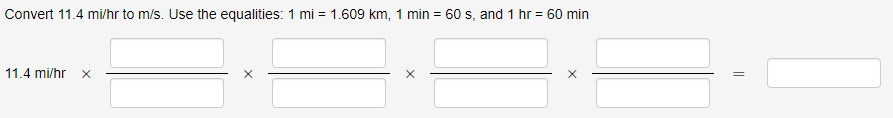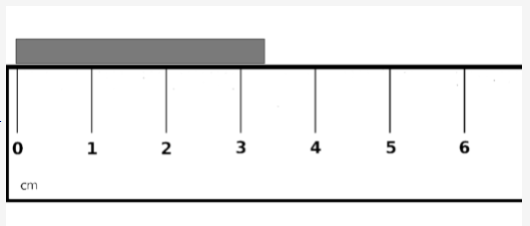## WeBWorK Main Forum

### WeBWorK for Chemistry, Significant Figures, Dimensional Analysis, more...### WeBWorK for Chemistry, Significant Figures, Dimensional Analysis, more...

by Lee McPherson -
Number of replies: 4

My name is Lee McPherson and I'm a part-time chemistry instructor at Portland Community College.  I've been working on software for both chemistry and general amusement for several different platforms for a little while.  However, I had always been interested in making software that can understand significant figures and do math operations with them.  It started in C# but I have since discovered the WeBWorK platform and decided to use it instead.  All of this work is at the following github repo:  limefrogyank/WeBWorKChemistry: WeBWorK scripts for doing problems related to Chemistry (and beyond!) (github.com)

I'm posting this now, because I would seriously love some help expanding this content and testing it.  We're slowly building some course content using these tools and discovering flaws to fix, but hope to have it up and running by winter term.

InexactValue

You can create an InexactValue with a string:

InexactValue("2.00");

You can create one with a number and explicit number of sig figs:

InexactValue(2, 3);  # essentially creates the value 2.00

You can even explicitly make the value exact.  (This is useful when you want to use the methods on InexactValue.  It will also be useful later with units.)

InexactValue(2, Infinity);

The methods include getting the number of sig figs.  This is useful for questions asking a student how many significant figures a number might have:

$inexactValue->sigFigs Best of all, you can do math with InexactValue and the correct significant figures are calculated according to the standard rules taught in most chemistry courses. $result = InexactValue("4.00") * InexactValue("2.0");# $result contains an InexactValue with value of 8 and 2 significant figures. The string output will be "8.0".  InexactValueWithUnits This is an extension of InexactValue with a modified version of the units macro. To make an InexactValueWithUnits, you provide exactly what you would provide a normal InexactValue as an array for the first parameter, then the units for the second parameter. $n1 = InexactValueWithUnits('1.609', 'km');$d1 = InexactValueWithUnits(['1', Infinity], 'mi'); When you do math with these values, the units automatically combine correctly: $result = $n1/$d1;# using values above# $result contains an InexactValueWithUnits that will output the string: 1.609 km mi^-1 Continuing in next reply...In reply to Lee McPherson ### Re: WeBWorK for Chemistry, Significant Figures, Dimensional Analysis, more... by Lee McPherson - ParserDimensionalAnalysis This is a utility that I wrote to enable easier problem writing when you want students to show how they did a conversion using dimensional analysis. It uses parser::MultiAnswer as a base. As an example, let's assume you want the student to do a four-step dimensional analysis problem to arrive at an answer. This is what the problem looks like to the student:To see how to format this for display and for hardcopy, see the demo section for dimensional analysis. Since we want the student to fill in the rest, those are answer blanks that need to be graded. While optional, you might also want to weight the final answer a little more than the work to get that answer. You would simply setup the problem like this: # The dimensional analysis part$ma = MultiAnswer($n1,$d1,$n2,$d2,$n3,$d3,$n4,$d4,$answer)->asDimensionalAnalysis($given);
# This weights the final answer at 50% and splits the rest among the conversion factors.
my $finalAnswerWeight = 50; my$remain = 100-$finalAnswerWeight; my @ansArr =$ma->cmp();
my $finalAns = pop @ansArr; for ($i=0; $i<scalar @ansArr;$i++){
WEIGHTED_ANS($ansArr[$i], $remain/(scalar @ansArr)); # sets weighting for dimensional analysis blanks } WEIGHTED_ANS($finalAns, $finalAnswerWeight); # sets weighting for final answer So what is asDimensionalAnalysis doing? First, it distinguishes the numerators and denominators as pairs that go together. But the order does NOT matter. The final parameter in the MultiAnswer part is always the answer. asDimensionalAnalysis will recalculate the student's answer using their dimensional analysis and compare it to the student's provided answer.In reply to Lee McPherson ### Re: WeBWorK for Chemistry, Significant Figures, Dimensional Analysis, more... by Lee McPherson - InexactValue has an internal tolerance that is 0 by default and uses absolute values (as opposed to relative) by default as well. If you are having students measure a value using an analog device, i.e. a graduated cylinder with liquid in it, you will get a few different answers that are all acceptable. For example, if you were measuring a block against a ruler like this:A student could write 3.2, 3.3, 3.4, or 3.5 as the answer, especially if viewing the problem on a tiny phone screen. Therefore, you could set the answer as 3.3 but add a 0.2 tolerance as well. Since tolerance is specific to the value, not the entire context, you need to set the tolerance via a final hash parameter. $m0 = InexactValue(3.3, 2, { tolerance => 0.2});

There is also a method called simpleUncertainty that outputs the uncertainty value for an intro-level chemistry problem. (i.e. +/- 1 for the last significant digit). If you would like to help develop a proper uncertainty value (i.e. adding absolute uncertainty and multiplying/dividing relative uncertainty), please open an issue and start submitting PRs!